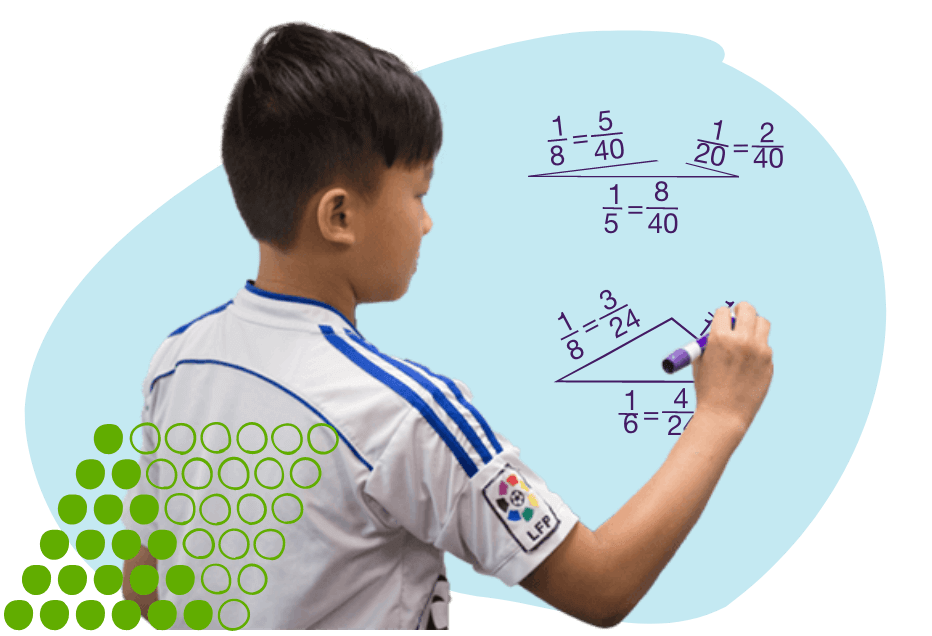# Live Math Classes with Top InstructorsSince 1993, Art of Problem Solving has helped train the next generation of intellectual leaders. Hundreds of thousands of our students have gone on to attend prestigious universities, win global math competitions, and achieve success in highly competitive careers.Rigorous Math Curriculum
Designed by PhDs from MIT, Princeton, and Stanford, our curriculum has helped thousands of motivated students develop the problem-solving skills they need to succeed in school and beyond.At-Home Learning Solution
Whether you need a comprehensive math curriculum or after-school enrichment, Virtual Campus fits the bill. Through small classes, students master key skills, such as critical thinking and problem solving.Fully Engaged Students
With a blend of a video-based live classroom, captivating instructors, and an interactive comic-style interface for the elementary levels, your student will be fully absorbed in our virtual content.

## Year-Round Math

For motivated students in Grades 2-8Ages: 7-9

### Honors Math 2

Build number sense by learning place value, number line, addition and subtraction, practice estimation and pattern recognition.Ages: 8-10

### Honors Math 3

Explore perimeter and area, dive deep into multiplication and division, introduce fractions and the use of variables.Ages: 9-11

### Honors Math 4

Expand knowledge of numbers to negative integers, decimals and binary numbers, study angles and symmetry, introduce Venn Diagrams and probability.Ages: 10-12

### Honors Math 5

Study 3D shapes, explore factors, ratios, square roots and exponents, solve word problems by creating and solving symbolic equations.Ages: 11-13

### Honors Math 6

Synthesize the fundamentals of arithmetic, explore geometric tools and strategies, introduce basic concepts in discrete math and statistics.Ages: 11-13

### Contest Math 6

Cover the topics in Honors Math 6, plus additional math competition problems from MATHCOUNTS and the MAA AMC 8.Ages: 12–14

### Honors Math 7

Master algebra topics such as linear and quadratic functions, exponents and logarithms, sequences and series, complex numbers, and more!Ages: 12–14

### Contest Math 7

Cover the topics in Honors Math 7, plus additional math competition problems and mock contests from MATHCOUNTS and the MAA AMC 8.Ages: 7-9

### Honors Math 2

Build number sense by learning place value, number line, addition and subtraction, practice estimation and pattern recognition.Ages: 8-10

### Honors Math 3

Explore perimeter and area, dive deep into multiplication and division, introduce fractions and the use of variables.Ages: 9-11

### Honors Math 4

Expand knowledge of numbers to negative integers, decimals and binary numbers, study angles and symmetry, introduce Venn Diagrams and probability.Ages: 10-12

### Honors Math 5

Study 3D shapes, explore factors, ratios, square roots and exponents, solve word problems by creating and solving symbolic equations.Ages: 11-13

### Honors Math 6

Synthesize the fundamentals of arithmetic, explore geometric tools and strategies, introduce basic concepts in discrete math and statistics.Ages: 11-13

### Contest Math 6

Cover the topics in Honors Math 6, plus additional math competition problems from MATHCOUNTS and the MAA AMC 8.Ages: 12–14

### Honors Math 7

Master algebra topics such as linear and quadratic functions, exponents and logarithms, sequences and series, complex numbers, and more!Ages: 12–14

### Contest Math 7

Cover the topics in Honors Math 7, plus additional math competition problems and mock contests from MATHCOUNTS and the MAA AMC 8.

## Go Above and Beyond with AoPS

AoPS Academy’s highly engaging curriculum has helped hundreds of thousands of motivated students leapfrog way ahead of most standard math curricula.### Collaborate with exceptional classmates from across the country## Why Students & Parents  AoPS Academy“My son LOVES his class! The math is inspiring, the teacher has a wonderful sense of humor, and he got to meet other students who love math as much as he does. Amazing job! Thank goodness we found AoPS!”Sign In